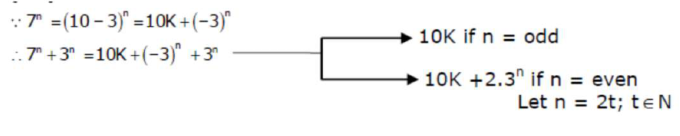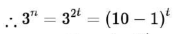# The total number of two digit numbersQuestion:

The total number of two digit numbers 'n', such that $3^{n}+7^{n}$ is a multiple of 10 , is

Solution:$=10 p+(-1)^{t}$

$=10 p \pm 1$

$\therefore$ if $\mathrm{n}=$ even then $7^{n}+3^{n}$ will not be multiply of 10

So if $n$ is odd then only $7^{n}+3^{n}$ will be multiply of 10

$\therefore \mathrm{n}=11,13,15, \ldots \ldots, \ldots, 99$

$\therefore$ Ans 45